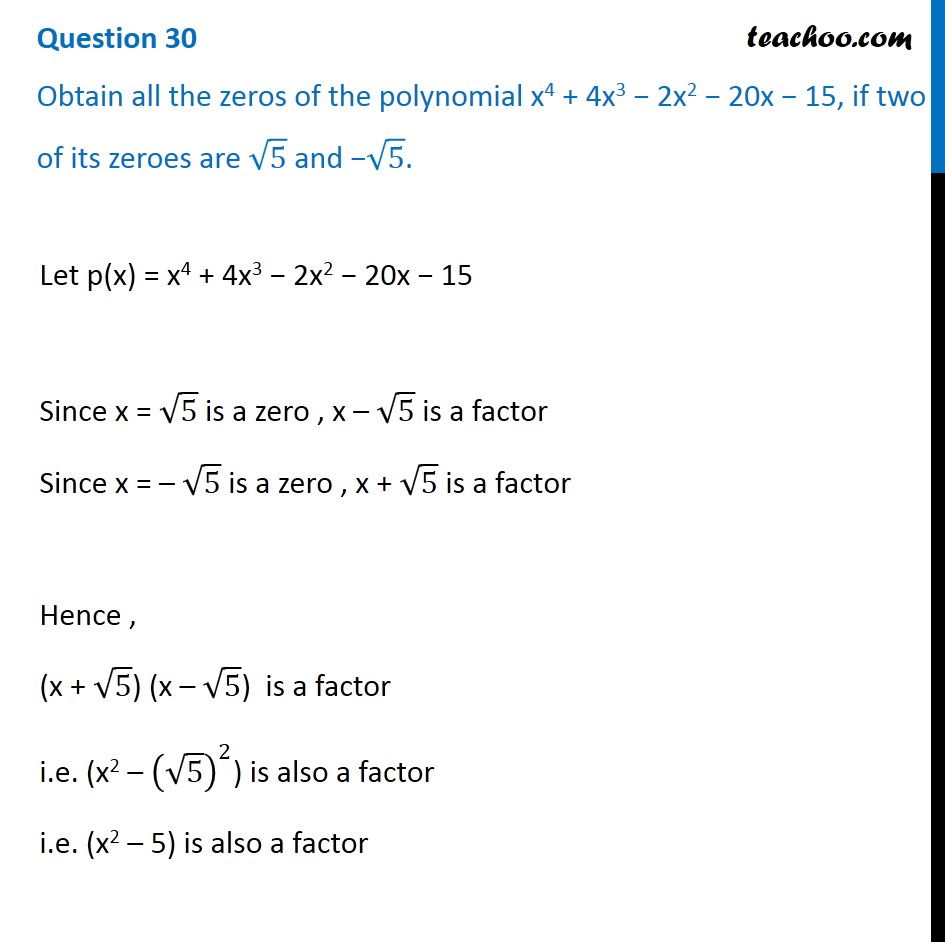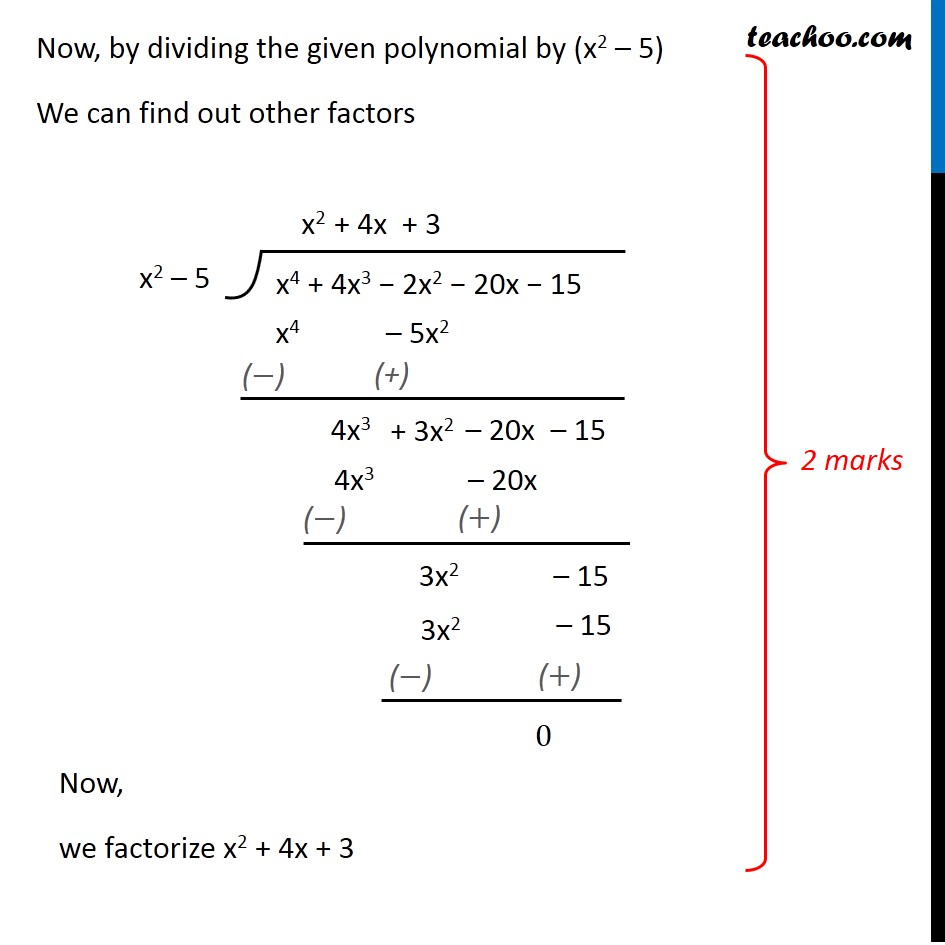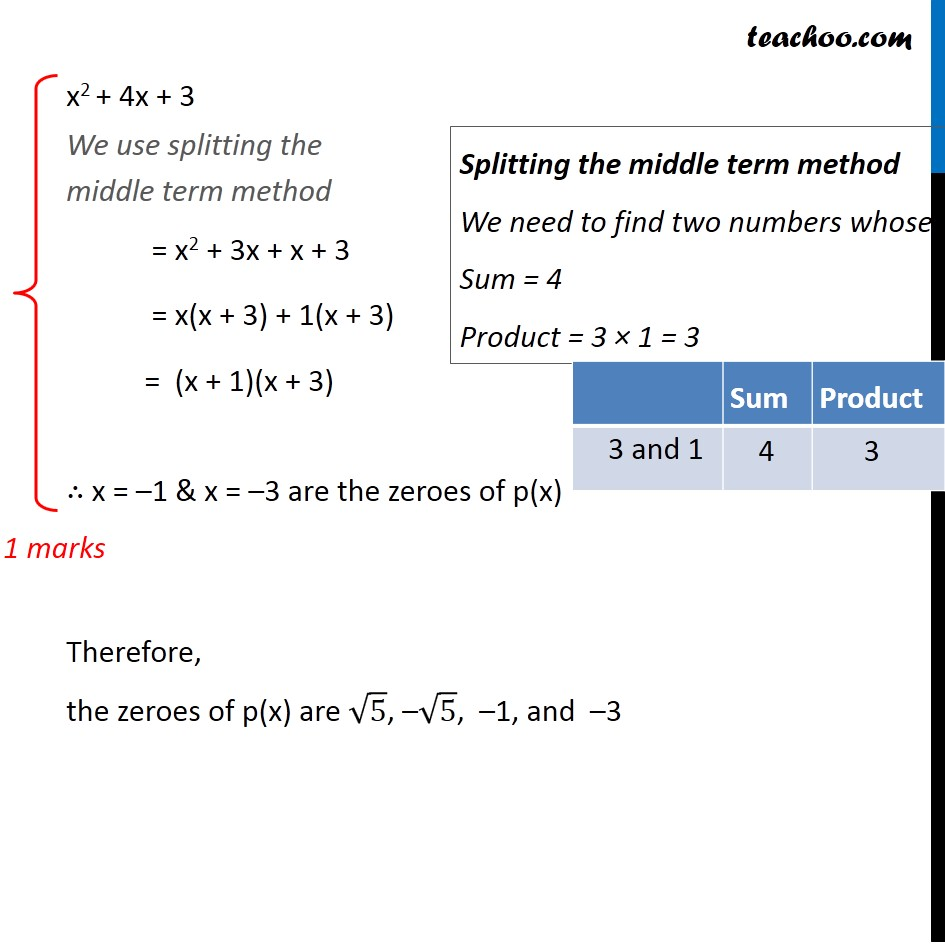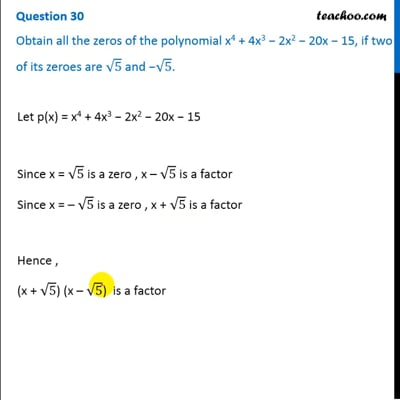CBSE Class 10 Sample Paper for 2020 Boards - Maths Standard

Class 10
Solutions of Sample Papers for Class 10 Boards

## Obtain all the zeros of the polynomial x 4 + 4x 3 − 2x 2 − 20x − 15, if two of its zeroes are √5 and −√5.This video is only available for Teachoo black users

Note : This is similar to Example 9 of NCERT – Chapter 2 Class 10

### Transcript

Question 30 Obtain all the zeros of the polynomial x4 + 4x3 − 2x2 − 20x − 15, if two of its zeroes are √5 and −√5. Let p(x) = x4 + 4x3 − 2x2 − 20x − 15 Since x = √5 is a zero , x – √5 is a factor Since x = – √5 is a zero , x + √5 is a factor Hence , (x + √5) (x – √5) is a factor i.e. (x2 – (√5)^2) is also a factor i.e. (x2 – 5) is also a factor Now, by dividing the given polynomial by (x2 – 5) We can find out other factors Now, we factorize x2 + 4x + 3 x2 + 4x + 3 We use splitting the middle term method = x2 + 3x + x + 3 = x(x + 3) + 1(x + 3) = (x + 1)(x + 3) Splitting the middle term method We need to find two numbers whose Sum = 4 Product = 3 × 1 = 3 ∴ x = –1 & x = –3 are the zeroes of p(x) Therefore, the zeroes of p(x) are √5, –√5, –1, and –3